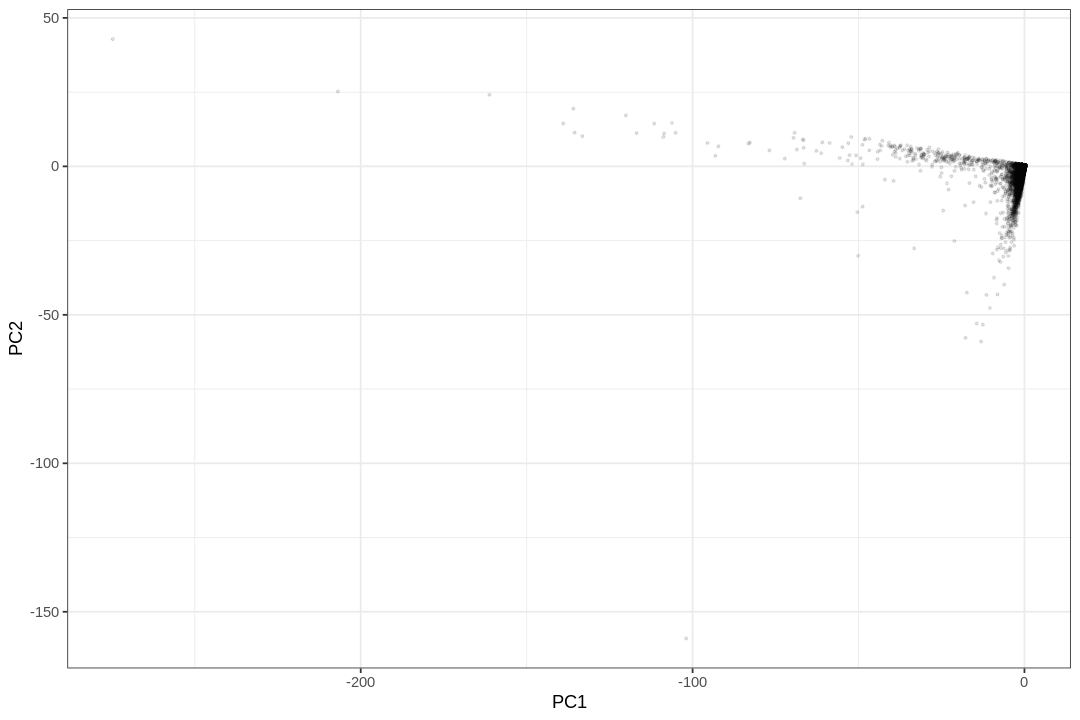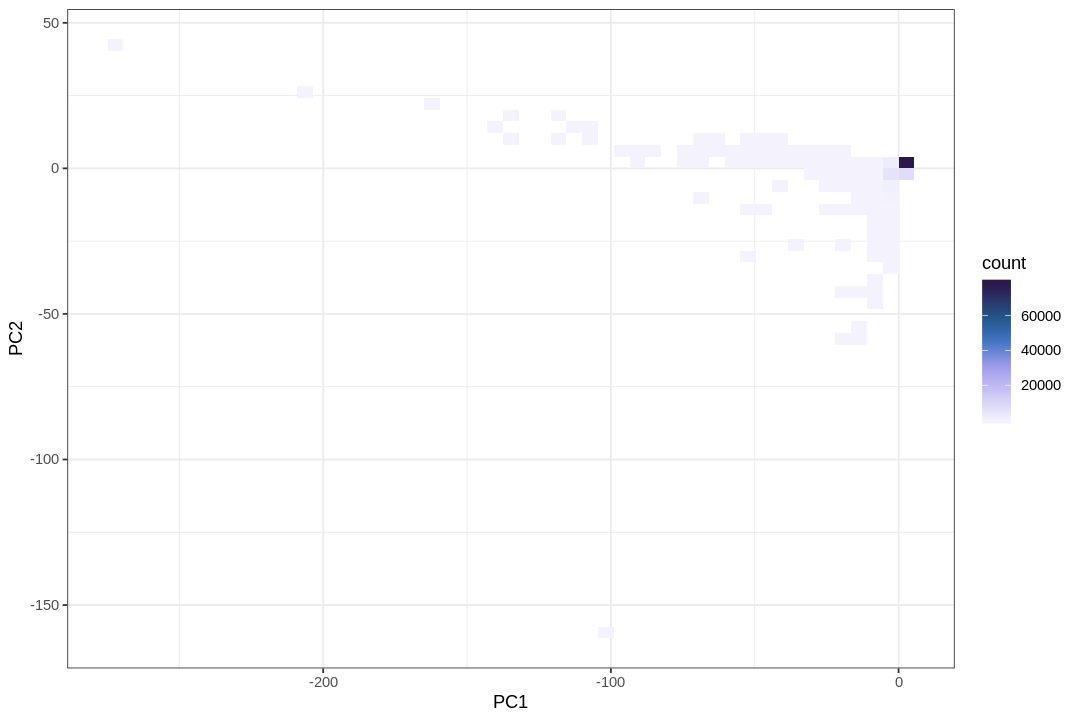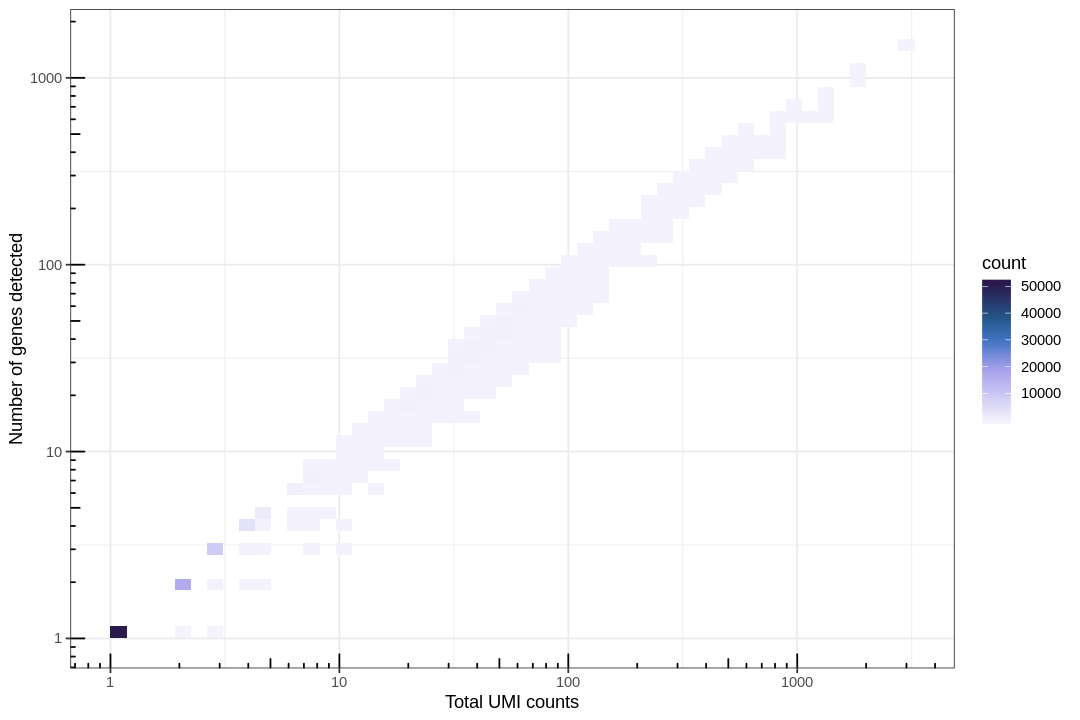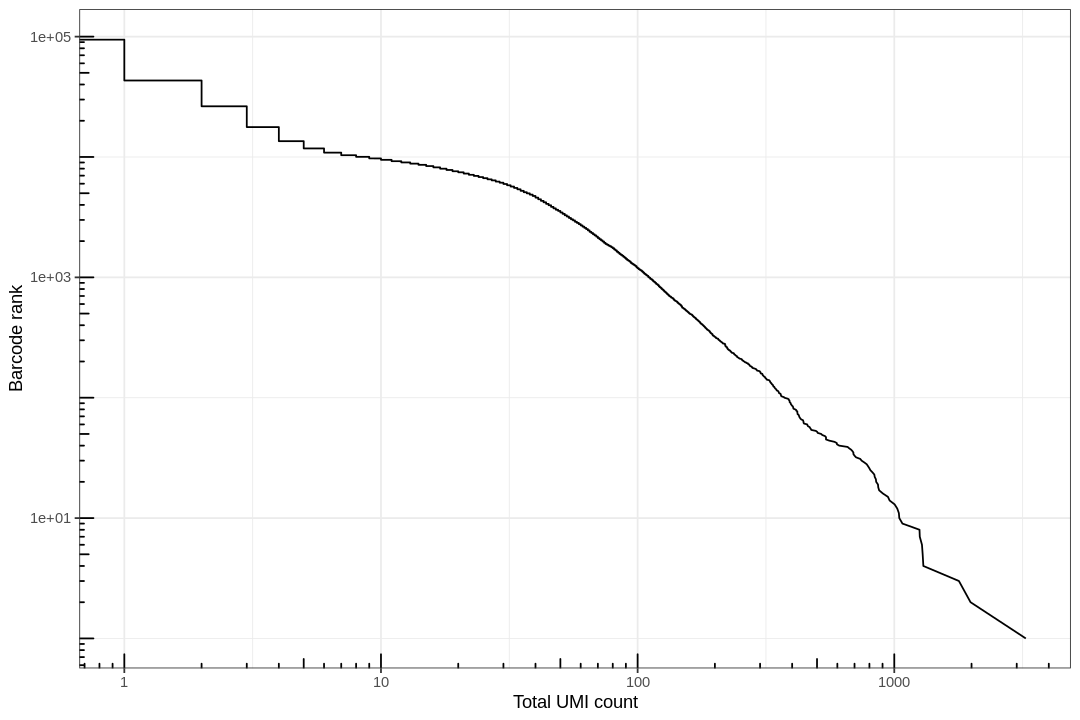# Introduction to single-cell RNA-seq I: pre-processing and quality control¶

This R notebook demonstrates the use of the kallisto and bustools programs for pre-processing single-cell RNA-seq data (also available as a Python notebook). It streams in 1 million C. elegans reads, pseudoaligns them, and produces a cells x genes count matrix in about a minute. The notebook then performs some basic QC. It expands on a notebook prepared by Sina Booeshaghi for the Genome Informatics 2019 meeting, where he ran it in under 60 seconds during a 1 minute "lightning talk".

The kallistobus.tools tutorials site has a extensive list of follow-up tutorials and vignettes on single-cell RNA-seq.

 ```1 2 3 4 5``` ``````#@title install.packages("vembedr") library("htmltools") library("vembedr") embed_youtube("x-rNofr88BM", width=560, height=315) ``````
``````Installing package into ‘/usr/local/lib/R/site-library’
(as ‘lib’ is unspecified)
``````

``````</head>
<body>
<iframe src="https://www.youtube.com/embed/x-rNofr88BM" width="560" height="315" frameborder="0" allowfullscreen=""></iframe>
</body>
``````

The notebook was written by A. Sina Booeshaghi, Lambda Lu and Lior Pachter. If you use the methods in this notebook for your analysis please cite the following publication, on which it is based:

• Melsted, P., Booeshaghi, A.S. et al. Modular and efficient pre-processing of single-cell RNA-seq. bioRxiv (2019). doi:10.1101/673285

## Setup¶

 ```1 2``` ``````# This is used to time the running of the notebook start_time <- Sys.time() ``````

### Install R packages¶

 `1` ``````install.packages(c("irlba", "scico"), Ncpus = 2) ``````
``````Installing packages into ‘/usr/local/lib/R/site-library’
(as ‘lib’ is unspecified)
``````
 ```1 2 3 4 5 6``` ``````library(Matrix) library(irlba) library(ggplot2) # Tidyverse is pre-installed, yay! library(dplyr) library(scico) theme_set(theme_bw()) ``````

### Install kb-python (includes kallisto and bustools)¶

 `1` ``````system("pip3 install kb-python") ``````

 ```1 2 3 4 5 6 7 8``` ``````# The quantification of single-cell RNA-seq with kallisto requires an index. # Indices are species specific and can be generated or downloaded directly with `kb`. # Here we download a pre-made index for C. elegans (the idx.idx file) along with an auxillary file (t2g.txt) # that describes the relationship between transcripts and genes. download.file("https://caltech.box.com/shared/static/82yv415pkbdixhzi55qac1htiaph9ng4.idx", destfile = "idx.idx") download.file("https://caltech.box.com/shared/static/cflxji16171skf3syzm8scoxkcvbl97x.txt", destfile = "t2g.txt") ``````

## Pseudoalignment and counting¶

In this notebook we pseudoalign 1 million C. elegans reads and count UMIs to produce a cells x genes matrix. These are located at XXX and instead of being downloaded, are streamed directly to the Google Colab notebook for quantification. See this blog post for more details on how the streaming works.

The data consists of a subset of reads from GSE126954 described in the paper:

### Run kallisto and bustools¶

 ```1 2``` ``````system("kb count -i idx.idx -g t2g.txt --overwrite -t 2 -x 10xv2 https://caltech.box.com/shared/static/fh81mkceb8ydwma3tlrqfgq22z4kc4nt.gz https://caltech.box.com/shared/static/ycxkluj5my7g3wiwhyq3vhv71mw5gmj5.gz", intern = TRUE) ``````

## Basic QC¶

### Represent the cells in 2D¶

 ```1 2``` ``````# Read in the count matrix that was output by `kb`. mat <- readMM("/content/counts_unfiltered/cells_x_genes.mtx") ``````
 ```1 2``` ``````# Convert to dgCMatrix, which is a compressed, sparse matrix format mat <- as(mat, "dgCMatrix") ``````
 `1` ``````dim(mat) ``````
1. 95372
2. 22113

Here cells are in rows and genes are in columns, while usually in single cell analyses, cells are in columns and genes are in rows. Here most "cells" are empty droplets. What if we do PCA now?

 ```1 2 3``` ``````# Perform PCA pca_res <- prcomp_irlba(mat, n = 2) # scales and centers by default pca_x <- as.data.frame(pca_res\$x) ``````
 ```1 2 3``` ``````# Plot the cells in the 2D PCA projection ggplot(pca_x, aes(PC1, PC2)) + geom_point(alpha = 0.1, size = 0.5) ``````While the PCA plot shows the overall structure of the data, a visualization highlighting the density of points reveals a large number of droplets represented in the lower left corner.

 ```1 2 3``` ``````ggplot(pca_x, aes(PC1, PC2)) + geom_bin2d(bins = 50) + scale_fill_scico(palette = "devon", direction = -1, end = 0.95) ``````The following plot helps clarify the reason for the concentrated points in the lower-left corner of the PCA plot.

### Test for library saturation¶

 ```1 2``` ``````df2 <- tibble(nCount = rowSums(mat), nGene = rowSums(mat > 0)) ``````
 ```1 2 3 4 5``` ``````ggplot(df2, aes(nCount, nGene)) + geom_bin2d(bins = 50) + scale_fill_scico(palette = "devon", direction = -1, end = 0.95) + scale_x_log10() + scale_y_log10() + annotation_logticks() + labs(x = "Total UMI counts", y = "Number of genes detected") ``````
``````Warning message:
“Transformation introduced infinite values in continuous x-axis”
Warning message:
“Transformation introduced infinite values in continuous y-axis”
Warning message:
“Removed 1018 rows containing non-finite values (stat_bin2d).”
``````Here we see that there are a large number of near empty droplets. A useful approach to filtering out such data is the "knee plot" shown below.

### Examine the knee plot¶

The "knee plot" was introduced in the Drop-seq paper: - Macosko et al., Highly parallel genome-wide expression profiling of individual cells using nanoliter droplets, 2015. DOI:10.1016/j.cell.2015.05.002

In this plot cells are ordered by the number of UMI counts associated to them (shown on the x-axis), and the fraction of droplets with at least that number of cells is shown on the y-axis:

 ``` 1 2 3 4 5 6 7 8 9 10 11 12``` ``````# Create the knee plot tot_counts <- rowSums(mat) df <- tibble(total = tot_counts, rank = row_number(desc(total))) %>% distinct() %>% arrange(rank) options(repr.plot.width=9, repr.plot.height=6) ggplot(df, aes(total, rank)) + geom_path() + scale_x_log10() + scale_y_log10() + annotation_logticks() + labs(y = "Barcode rank", x = "Total UMI count") ``````
``````Warning message:
“Transformation introduced infinite values in continuous x-axis”
`````````1 2``` ``````# An option is to filter the cells and genes by a threshold # mat_filtered <- mat[rowSums(mat) > 30, colSums(mat) > 0] ``````

### Exercises¶

• The "knee plot" is sometimes shown with the UMI counts on the y-axis instead of the x-axis, i.e. flipped and rotated 90 degrees. Make the flipped and rotated plot. Is there a reason to prefer one orientation over the other?
 ``` 1 2 3 4 5 6 7 8 9 10 11 12``` ``````# # Create the flipped and rotated knee plot # tot_counts <- rowSums(mat) # df <- tibble(total = tot_counts, # rank = row_number(desc(total))) %>% # distinct() %>% # arrange(rank) # # options(repr.plot.width=9, repr.plot.height=6) # ggplot(df, aes(rank, total)) + # geom_path() + # scale_y_log10() + scale_x_log10() + annotation_logticks() + # labs(y = "Total UMI count", x = "Barcode rank") ``````

For more information on this exercise see Rotating the knee (plot) and related yoga.

## Discussion¶

This notebook has demonstrated the pre-processing required for single-cell RNA-seq analysis. `kb` is used to pseudoalign reads and to generate a cells x genes matrix. Following generation of a matrix, basic QC helps to assess the quality of the data.

 ```1 2``` ``````# Running time of the notebook Sys.time() - start_time ``````
``````Time difference of 2.6641 mins
``````

Feedback: please report any issues, or submit pull requests for improvements, in the Github repository where this notebook is located.